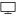# Linear Models and Regression

Lecture notes for the two-semester course "Linear Models and Regression I-II"

## Tabs

1. Introduction (Definition of linear models, examples)
2. Estimation of Parameters (Vector and matrix representation, estimation of $$\theta$$, estimation of $$\sigma$$, Gauss-Markov theorem and standard errors, performance of the estimators in case of misspecified models, parametrizations for categorical covariates)
3. Tests and Confidence Regions (Multivariate normal distributions, the joint distribution of $$\hat{\theta}$$ and $$\hat{\sigma}$$, student confidence intervalle and tests, F confidence regions and tests, further simultaneous confidence regions, non-central F distributions and approximation errors, calibration, random effects)
4. Regression diagnostics (Leverage, an application of the Central Limit Theorem, residual analyses, transformations)
5. Nonparametric Regression (Spline regression, local polynomials, regularization)
6. General Considerations about Estimation (Means and quantiles als optimal predictors, loss and risk functions, maximum likelihood estimation, application to regression problems)
7. Logistic Regression and Related Models (Logistic regression, general asymptotic considerations, methods for multicategorical response, Poisson regression, supplements)
8. Bootstrap procedures (Bootstrap for simple samples, bootstrap for regression)
9. Empirical Likelihood Procedures
10. Appendix: Various Auxiliary Results (QR decomposition, Iteratively reweighted least squares, mean vectors and covariance matrices, B-splines, weak convergence of distributions, Central Limit Theorem, couplings and Mallows distances, an inequality for sums of independent random vectors)

## Files### LinModpdf   7.4 MB   Version: 30   Today, 08:22   Page Count: 290